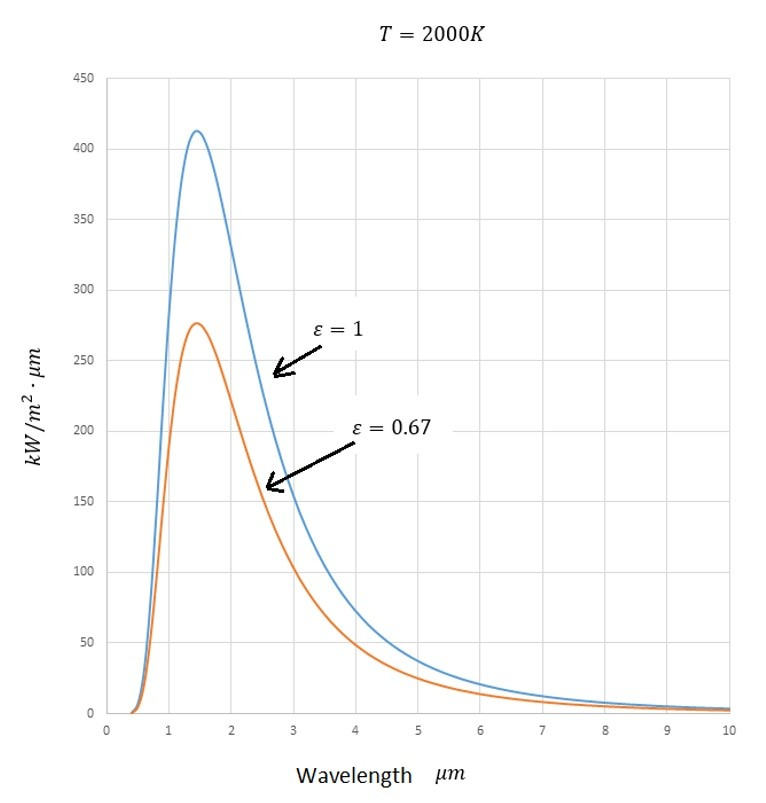# Black Body and Grey Body Theory

Radiation heat transfer is a type of electromagnetic radiation. This means that it will propagate at the speed of light, 3 x 108 m/s. This can be represent by the following equation.

(Eq 1)  $c = λν$

c = Speed of Light

λ = Wavelength

v = frequency

Thermal radiation will lie in the 0.1 to 100 micro-meter frequency range of the electromagnetic spectrum. Thermal energy propagates in a discrete quanta of energy which can be represented by the following equation.

(Eq 2) $E=hν=mc^2$

h = 6.625 X 10-34 J-s

In the above equation h represents Planck’s constant.

Notice that the above equation is related to Einstein’s equation E = mc2. Due to this fact equation 2 can be transformed into the equation below which uses quantum-statistical thermodynamics to determine the energy density of radiation per unit volume and per unit wave length.

(Eq 3) $u_λ=\frac{8πhcλ^-5}{e^{hc/λkt}-1}$

k = 1.38 X 10-23 J/molecule-K

In the above equation k is the Boltzmann constant.

Finally, when equation 3 is integrated over the entire thermal radiation frequency range the resulting equation will be the heat transfer equation for radiation heat transfer for a black body.

(Eq 4) $E_b=σT^4$

σ = 5.669 X 10-8 W/m2-K4

A black body is an object that will theoretically absorb all thermal radiation. A black body is called a black body because generally they appear black to the eye since they do not reflect radiation. However, this is not always the case. Snow and ice, which appear white to the eye, are actually black bodies for long-wavelength thermal radiation. The same thing applies to a variety of white paints.

Now as mentioned above a black body will absorb all thermal radiation. However, if the body is a grey body it will reflect a certain amount of the thermal energy back into space, or potential back at the radiating body that the thermal radiation came from. Finally, it is also possible that an object might be transmitting its own thermal radiation. There are three ways that thermal radiation can interact with an object. Thermal radiation could be absorbed, reflected, or transmitted. The total summation of all of these radiation types should sum to 1 as seen in the equation below.

(Eq 5) $ρ+α+τ=1$

## Emissivity

The ability for a body to emit thermal radiation is known as the emissivity of the body. When the emissivity is taken into consideration equation 4 will be modified to the following.

(Eq 6)  $E=εσT^4$

The emissivity of a black body will be 1. To calculate emissivity of a grey body the following equation would be used.

(Eq 7) $ε=\frac{E}{E_b}$

## Energy Transmitted Wave Length

Finally, as mentioned before, thermal radiation is transmitted over a wavelength range of 0.1-100 micro-meters within the electromagnetic spectrum. The amount of energy that is emitted however is dependent on the wavelength of interest within that frequency range. To determine the energy emitted for a given wavelength the equation below would be used.

(Eq 8) $E_λ=ε\frac{C_1λ^-5}{e^{c_2/λT}-1}$

C1 = 3.743 X 108 W-μm4/m2

C2 = 1.4387 X 104μm-K

Refer to the image below to see how the emitted energy changes as the wavelength changes.From the above image it can be seen that most of the energy transmitted by thermal radiation happens between 1 and 4 micro meters.